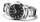Minute hand v2

In how many minutes describe the minute hand angle 60 degrees?

Result

t =  10 min

Solution:Leave us a comment of example and its solution (i.e. if it is still somewhat unclear...):Be the first to comment!To solve this example are needed these knowledge from mathematics:

Need help calculate sum, simplify or multiply fractions? Try our fraction calculator.

Next similar examples:

1. MinutesWrite as fraction in basic form which part of the week is 980 minutes.
2. DoctorsIn the city operates 171 doctors. The city has 128934 citizens. How many citizens are per one doctor?
3. The resultHow many times I decrease the number 1632 to get the result 24?
4. Math classificationIn 3A class are 27 students. One-third got a B in math and the rest got A. How many students received a B in math?
5. Fractions 4How many 2/3s are in 6?
6. CakesOn the bowl were a few cakes. Jane ate one-third of them, Dana ate a quarter of those cakes that remained. a) What part (of the original number of cakes) Dana ate? b) At least how many cakes could be (initially) on thebowl?
7. Lengths of the poolMiguel swam 6 lengths of the pool. Mat swam 3 times as far as Miguel. Lionel swam 1/3 as far as Miguel. How many lengths did mat swim?
8. Angles 1It is true neighboring angles have not common arm?
9. Pizza 5You have 2/4 of a pizza and you want to share it equally between 2 people how much pizza does each person get?
10. Cake 71/3 of a cake shared with 4 people. What share of the whole cake has each people?
11. Product of two fractionsProduct of two fractions is 9 3/5 . If one of the fraction is 9 3/7. Find the other fraction.
12. In fractionsAn ant climbs 2/5 of the pole on the first hour and climbs 1/4 of the pole on the next hour. What part of the pole does the ant climb in two hours?Added together and write as decimal number: LXVII + MLXIVWrite the fraction 3/22 as a decimal.Write as a fraction and a decimal. One and two plus three and five hundredthsWrite the mixed number as an improper fraction. 166 2/3Zdeněk picked up 15 l of water from a 100-liter full-water barrel. Write a fraction of what part of Zdeněk's water he picked.